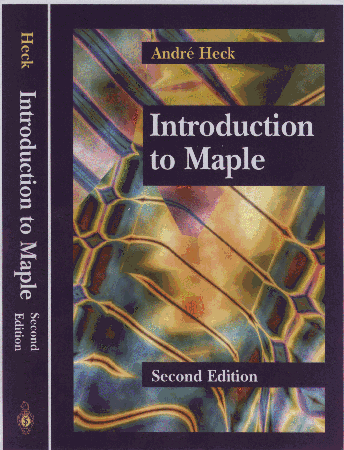# Introduction to Maple

### 2nd Edition### ISBN 0-387-94535-0

700 pp, 190 figs., 48 tables.

## About the Revised and Expanded Edition

The 2nd edition of the book is fully revised and updated to Maple V Release 4. Hardly any chapter has been left untouched, but substantially changed or new chapters are: the assume facility, I/O, integration, composite data types, simplification, graphics, differential equations, and matrix algebra. Tables summarize features, command options, etc., and constitute a quick reference. Many new examples have been included that show how to use Maple as a problem solver, how to assist the system during computations, and how to extend its built-in facilities.

## Contents

Completely new sections are emphasized below.

• Preface to First Edition
• Preface to Second Edition
1. Introduction to Computer Algebra
1. What is Computer Algebra?
2. Computer Algebra Systems
3. Some Properties of Computer Algebra Systems
4. Advantages of Computer Algebra
5. Limitations of Computer Algebra
6. Design of Maple

2. The First Steps: Calculus on Numbers
1. Getting Started
2. Getting Help
3. Integers and Rational Numbers
4. Irrational Numbers and Floating-Point Numbers
5. Algebraic Numbers
6. Complex Numbers
7. Exercises

3. Variables and Names
1. Assignment and Unassignment
2. Evaluation
3. Names of Variables
4. Basic Data Types
5. Attributes
6. Properties
7. Exercises
8. Commonly Used Surface Data Types

4. Getting Around with Maple
1. Maple Input and Output
2. The Maple Library
3. Reading and Writing Files
4. Importing and Exporting Numerical Data
5. Low-Level I/O
6. Code Generation
7. Changing Maple to your own Taste
8. Exercises

5. Polynomials and Rational Functions
1. Univariate Polynomials
2. Multivariate Polynomials
3. Rational Functions
4. Conversions
5. Exercises

6. Internal Data Representation and Substitution
1. Internal Representation of Polynomials
2. Generalized Rational Expressions
3. Substitution
4. Exercises

7. Manipulation of Polynomials and Rational Expressions
1. Expansion
2. Factorization
3. Canonical Form and Normal Form
4. Normalization
5. Collection
6. Sorting
7. Exercises

8. Functions
1. Mathematical Functions
2. Arrow Operators
3. Piecewise Defined Functions
4. Maple Procedures
5. Recursive Procedure Definitions
6. unapply
7. Operations on Functions
8. Anonymous Functions
9. Exercises

9. Differentiation
1. Symbolic Differentiation
2. Automatic Differentiation
3. Exercises

10. Integration and Summation
1. Indefinite Integration
2. Definite Integration
3. Numerical Integration
4. Integral Transforms
5. Assisting Maple's Integrator
6. Summation
7. Exercises

11. Series, Approximation, and Limits
1. Truncated Series
2. Approximation of Functions
3. Power Series
4. Limits
5. Exercises

12. Composite Data Types
1. Sequence
2. Set
3. List
4. Array
5. Table
6. Last Name Evaluation
7. Function Call
8. Conversion between Composite Data Types
9. Exercises

13. The Assume Facility
1. The Need of an Assume Facility
2. Basics of assume
3. An Algebra of Properties
4. Implementation of assume
5. Exercises
6. Hierarchy of Properties

14. Simplification
1. Automatic Simplification
2. expand
3. combine
4. simplify
5. convert
6. Trigonometric Simplification
7. Simplification w.r.t. Side Relations
8. Control over Simplification
9. Defining Your Own Simplification Routines
10. Exercises
11. Simplification Chart

15. Graphics
1. Some Basic Two-Dimensional Plots
2. Options of plot
3. The Structure of Two-Dimensional Graphics
4. The plottools Package
5. Special Two-Dimensional Plots
6. Two-dimensional Geometry
7. Plot Aliasing
8. A Common Mistake
9. Some Basic Three-Dimensional Plots
10. Options of plot3d
11. The Structure of Three-Dimensional Graphics
12. Special Three-Dimensional Plots
13. Data Plotting
14. Animation
15. List of Plot Options
16. Exercises.

16. Solving Equations
1. Equations in One Unknown
2. Abbreviations in solve
3. Some Difficulties
4. Systems of Equations
5. The Gröbner Basis Method
6. Inequalities
7. Numerical Solvers
8. Other Solvers in Maple
9. Exercises

17. Differential Equations
1. First Glance at ODEs
2. Analytic Solutions
3. Taylor Series Method
4. Power Series Method
5. Numerical Solutions
6. DEtools
7. Perturbation Methods
8. Partial Differential Equations
9. Lie Point Symmetries of PDEs
10. Exercises

18. Linear Algebra: The linalg Package
2. Creating New Vectors and Matrices
3. Vector and Matrix Arithmetic
4. Basic Matrix Functions
5. Structural Operations
6. Vector Operations
7. Standard Forms of Matrices
8. Exercises.

19. Linear Algebra: Applications
1. Kinematics of the Stanford Manipulator
2. A 3-Compartment Model of Cadmium Transfer
3. Molecular-orbital Hückel Theory
4. Vector Analysis
5. Moore-Penrose Inverse
6. Exercises
• References
• Index